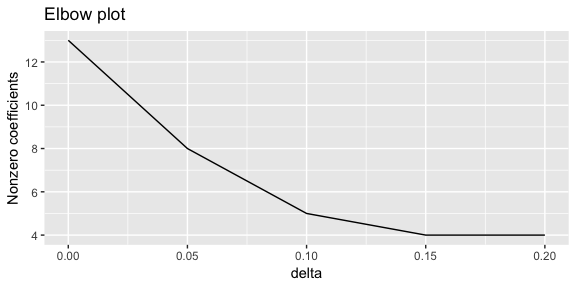# The hdme package: regression methods for high-dimensional data with measurement error

#### 2019-04-12

# Load the hdme package
library(hdme)

The hdme package contains algorithms for regression problems with measurement error when the number of covariates $$p$$ is on the same order as the number of samples $$n$$, or even larger.

# Measurement Error in Regression

## Classical Measurement Error Problems

Consider a linear regression model, $$y = X\beta + \epsilon$$, where $$y$$ is an $$n$$-dimensional response vector, $$X$$ is an $$n \times p$$ design matrix, and $$\epsilon$$ is normally distributed error. With $$n>p$$ (and $$X$$ positive definite), unbiased regression coefficients are obtained as

$\hat{\beta} = (X^{T}X )^{-1} X^{T} y.$

In many cases, however, the true covariates $$X$$ are not directly observed. Instead, we have noisy measurements

$W = X + U,$

where $$W$$ is the $$n\times p$$ measurement matrix, and $$U$$ is the $$n\times p$$ matrix of measurement errors. Assume for the moment that the $$n$$ rows of $$U$$, $$u_{i}$$ are identically and independently distributed with covariance matrix $$\Sigma_{uu}$$, i.e.,

$u_{i} \sim N(0, \Sigma_{uu}), ~ i = 1,\dots,n.$

Since we do not have measurements of $$X$$, using a classical linear regression model now yields coefficient estimates

$\hat{\beta}_{naive} = (W^{T}W )^{-1} W^{T} y,$ which is referred to as the naive estimate in the measurement error literature. Naive estimates are typically biased. For example, when all measurement errors are uncorrelated and each have variance $$\sigma_{u}$$, i.e., $$\Sigma_{uu} = \sigma_{u} I_{p\times p}$$, the expected value of $$\hat{\beta}$$ is

$E(\hat{\beta}_{naive}) = \frac{\sigma_{x}^{2}}{\sigma_{u}^{2} + \sigma_{x}^2} \beta = \gamma \beta,$ where $$\gamma$$ is the attenuation factor. Unbiased estimates for the case of linear regression can be obtained by minimizing the corrected loss function $L_{corr}(\beta) = \|y - W\beta\|^{2} - \beta^{T}\Sigma_{uu} \beta.$ This $$L_{corr}(\beta)$$ is not always convex. If the Hessian $$W^{T}W - \Sigma_{uu}$$ is positive definite, $$L_{corr}(\beta)$$ is convex, and the estimates can be found using

$\hat{\beta}_{corr} = (W^{T}W - \Sigma_{uu} )^{-1} W^{T} y.$ Otherwise, iteration methods must be used to find a minimum of $$L(\beta)$$.

An estimate $$\hat{\Sigma}_{uu}$$ of $$\Sigma_{uu}$$ can be typically obtained with replicate measurements.

Similar results hold for generalized linear models, e.g., logistic and Poisson regression: Measurement error typically leads to bias in the naive estimates, and correction methods can be used to obtained unbiased estimates. We refer to the book Carroll et al. (2006) for a thorough introduction to measurement error modeling.

## Measurement Error in High-Dimensional Regression Problems

Now consider the same setup as in the previous section, but with high-dimensional data. That is, the number of covariates $$p$$ is either on the same order as $$n$$, or even larger than $$n$$. In this case, even in the absence of measurement error, regularization is typically needed (see, e.g., Chapter 3 of Hastie, Tibshirani, and Friedman (2009)).

### The lasso

The lasso (Tibshirani (1996)) performs $$L1$$-regularization, which induces sparsity. A popular R implementation can be found in the glmnet package (Simon et al. (2011)). For linear regression models, the lasso finds $$\hat{\beta}$$ minimizing the loss $L(\beta) = \|y - X \beta\|^{2} + \lambda \|\beta\|_{1},$ where $$\lambda$$ is a regularization parameter. The lasso can also be formulated as a constrained optimization problem, $\text{minimize } ~\|y - X\beta\|^{2}~ \text{ subject to }~ \|\beta\|_{1} \leq R,$ where $$R$$ is in a one-to-one relationship with $$\lambda$$.

A lot of effort has been put into understanding the statistical properties of the lasso, both in the classical $$p<n$$ case and in the high-dimensional $$p>n$$ case, summarized in Bühlmann and Geer (2011). The results are typically either probabilistic upper bounds on or asymptotic limits for

• Prediction error $$E(\|y_{new} - X_{new}\hat{\beta}\|)$$ where $$(X_{new}, y_{new})$$ are a new sample of data.
• Sum of squared estimation error $$\|\hat{\beta} - \beta\|_{2}^{2}$$ or sum of absolute estimation error $$\|\hat{\beta} - \beta\|_{1}$$
• Covariate selection: If true coefficients in the index set $$J \subseteq \{1, \dots, p\}$$ are nonzero, while the rest are zero, i.e., $$\beta_{J} \neq 0$$ and $$\beta_{J^{C}} = 0$$, under which conditions will the lasso recover the true set of relevant covariates while correctly setting the irrelevant covariates to zero?

The impact of measurement error in the lasso for linear models has been studied by Sorensen, Frigessi, and Thoresen (2015). The authors show that estimation of $$\hat{\beta}$$ in the asymptotic limit suffers the same bias as for a multivariate linear model described above. Consistent covariate selection, on the other hand, requires stricter conditions than in the case without measurement error.

Using the glmnet package, we can illustrate the impact of measurement error in a small experiment.

First we set up the parameters and generate random data. In order to repeat the procedure, we create the function create_example_data for doing this. For illustration purposes, we set the true coefficient vector to $$\beta = (-2, -1, 0.5, 1, 2, 0, \dots, 0)^{T}$$.

create_example_data <- function(n, p, s = 5, sdX = 1, sdU = 0.5,
sdEpsilon = 0.1, family = "gaussian") {
# Independent true covariates with mean zero and standard deviation sdX
X <- matrix(rnorm(n * p, sd = sdX), nrow = n, ncol = p)
# if Gaussian, response has standard deviation sdEpsilon, and zero intercept
# if binomial, response is binomial with mean (1 + exp(-X %*% beta))^(-1)
beta <- c(-2, -1, 0.5, 1, 2, rep(0, p - s))

if(family == "gaussian") {
# True coefficient vector has s non-zero elements and p-s zero elements
y <- X %*% beta + rnorm(n, sd = sdEpsilon)
} else if (family == "binomial") {
# Need an amplification in the binomial case
beta <- beta * 3
y <- rbinom(n, size = 1, prob = (1 + exp(-X %*% beta))**(-1))
}

# The measurements W have mean X and standard deviation sdU.
# We assume uncorrelated measurement errors
W <- X + matrix(rnorm(n * p, sd = sdU), nrow = n, ncol = p)

return(list(X = X, W = W, y = y, beta = beta, sigmaUU = diag(p) * sdU))
}

We then call the function to get the data and put the result in the list ll.

n <- 100
p <- 500
set.seed(1000)
ll <- create_example_data(n, p)

Next we run the lasso with cross-validation on the true covariates and on the noisy measurements. We pick the coefficient estimate at $$\lambda_{1se}$$, i.e., on standard error on the sparse side of the cross-validation minimum. This is the default of the coef function for objects of class cv.glmnet.

library(glmnet)
library(dplyr)
# Lasso with cross-validation on data without measurement error
fit1 <- cv.glmnet(ll$X, ll$y)
# Lasso with cross-validation on data with measurement error
fit2 <- cv.glmnet(ll$W, ll$y)
# Create a data frame with results ([-1] because we drop the intercept)
lassoEstimates <- tibble(
index = rep(1:p, times = 3),
beta = c(ll$beta, as.numeric(coef(fit1)[-1]), coef(fit2)[-1]), label = c(rep("True values", p), rep("No measurement error", p), rep("Measurement error", p)) ) By plotting all the estimated regression coefficients, we see that when the data are subject to measurement error, the number of false positives may increase. Note that this is not necessarily the case for all choices of parameters. library(ggplot2) ggplot(lassoEstimates, aes(x = index, y = beta, color = label)) + geom_point() + xlab("p") + theme(legend.title=element_blank()) + ggtitle("Measurement error leading to false positives")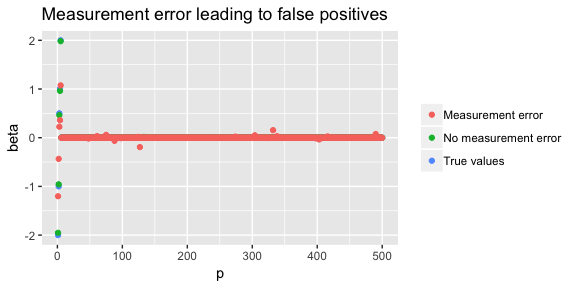We can also focus on the 5 parameters which are truly nonzero. In this case, we see that in the absence of measurement error, the lasso estimates values quite close to truth. With measurement error, on the other hand, the attenuation is quite clear: the estimates are biased toward zero. library(tidyr) estimatesOfNonzero <- lassoEstimates %>% spread(key = label, value = beta) %>% filter(True values != 0) %>% gather(key = label, value = beta, -index) ggplot(estimatesOfNonzero, aes(x = index, y = beta, color = label)) + geom_point() + xlab("p") + theme(legend.title=element_blank()) + ggtitle("Measurement error leading to attenuation")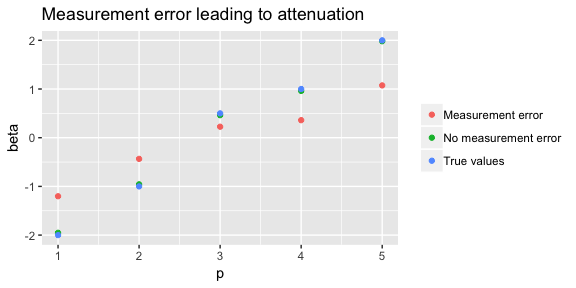### The Dantzig Selector The Dantzig selector Candes and Tao (2007) is closely related to the lasso. It is defined as the solution to the optimization problem $\text{minimize } ~ \|\beta\|_{1}, ~ \text{ subject to } ~ (1/n)\| X^{T} (y - X\beta)\|_{\infty} \leq \lambda,$ where $$\|\cdot\|_{\infty}$$ denotes the maximum component norm. An R implentation of the Dantzig selector can be found in the flare package Li et al. (2018). We illustrate it with the same data as in the previous section. Calling the slim method in the flare package yields the Dantzig selector solution for the default values of $$\lambda$$. We can compare the methods for data with and without measurement error. library(flare) #> Loading required package: lattice #> Loading required package: MASS #> #> Attaching package: 'MASS' #> The following object is masked from 'package:dplyr': #> #> select #> Loading required package: igraph #> #> Attaching package: 'igraph' #> The following object is masked from 'package:tidyr': #> #> crossing #> The following objects are masked from 'package:dplyr': #> #> as_data_frame, groups, union #> The following objects are masked from 'package:stats': #> #> decompose, spectrum #> The following object is masked from 'package:base': #> #> union # No measurement error, using X dantzig_fit_x <- slim(ll$X, ll$y, nlambda = 10, method = "dantzig", verbose = FALSE, lambda.min.ratio = 0.3) # Measurement error, using W dantzig_fit_w <- slim(ll$W, ll$y, nlambda = 10, method = "dantzig", verbose = FALSE, lambda.min.ratio = 0.3) Below is the regularization path for the Dantzig selector in the case of no measurement error. plot(dantzig_fit_x)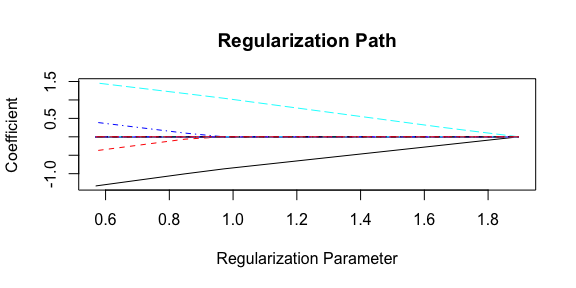Next is the regularization path for the Dantzig selector in the case of measurement error. plot(dantzig_fit_w)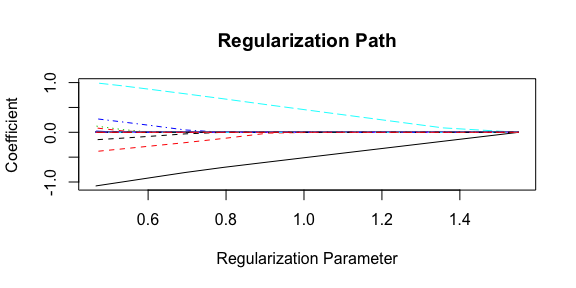A generalized Dantzig selector for generalized linear models was introduced by James and Radchenko (2009). It is defined as the solution to the optimization problem $\text{minimize } ~ \|\beta\|_{1}, ~ \text{ subject to } ~ (1/n)\| X^{T} (y - \mu(X\beta))\|_{\infty} \leq \lambda,$ where $$\mu(\cdot) \in \mathbb{R}^{n}$$ is the vector valued mean function of the generalized linear model. Examples include logistic regression with $$\mu(x) = (1+\exp(-x))^{-1}$$ and Poisson regression with $$\mu(x) = \exp(x)$$. Using an iterative reweighing algorithm, the generalized Dantzig selector can be solved as a sequence of linear optimization problems of the same form as the Dantzig selector for linear models. #### Generalized Dantzig Selector To our knowledge, no R package to date contains an implementation of the Generalized Dantzig Selector (GDS), although code is available on Professor Gareth James’ homepage. Since the Generalized Matrix Uncertainty Selector (GMUS) presented later in this vignette is a generalization of the GDS, we have included a function for computing the GDS, called fit_gds. # Number of samples n <- 1000 # Number of covariates p <- 50 # Create example data ll <- create_example_data(n, p, family = "binomial") Arguments to fit_gds. Only $$X$$ and $$y$$ are required. args(fit_gds) #> function (X, y, lambda = NULL, family = c("gaussian", "binomial")) #> NULL Fit the generalized Dantzig selector on the data. # Fit the Generalized Dantzig Selector gds_estimate <- fit_gds(ll$X, ll$y, family = "binomial") The result is a list of class gds. class(gds_estimate) #>  "gds" The list contains the intercept (which has not been penalized), the regression coefficients beta, the family, the value of lambda and the number of nonzero components of beta. By default, fit_gds uses the value $$\lambda_{min}$$ corresponding to the minimum cross-validated loss for the lasso, using cv.glmnet. str(gds_estimate) #> List of 5 #>$ intercept   : num 0.0447
#>  $beta : num [1:50] -3.964 -1.906 0.738 1.989 3.904 ... #>$ family      : chr "binomial"
#>  $lambda : num 0.00435 #>$ num_non_zero: num 12
#>  - attr(*, "class")= chr "gds"

At the moment, only a single value of lambda is accepted by GDS. We will fix this in the future, as well as adding a cross validation function.

# Corrected Lasso

## Corrected Lasso for Linear Regression

When an estimate of the measurement error covariance matrix $$\Sigma_{uu}$$ is available, a corrected lasso can be defined as minimizing the loss $\text{minimize } ~ L(\beta) = \|y - W \beta \|^{2} - \beta^{T} \Sigma_{uu} \beta + \lambda \|\beta\|_{1} ~\text{ subject to } \|\beta\|_{1} \leq R.$ Because we subtract the positive semidefinite matrix $$\Sigma_{uu}$$ from the convex function $$\|y - W\beta\|^{2}$$, this corrected lasso may be non-convex. In fact, when $$p>n$$ is is always non-convex. Hence, in order to avoid non-trivial solutions, we must constrain the solution to lie in some L1-ball with radius $$R$$. Since this problem involves two regularization parameters, $$\lambda$$ and $$R$$, it is more convenient to use the constrained version of the lasso

$\text{minimize } ~ L(\beta) = \|y - W \beta \|^{2} - \beta^{T} \Sigma_{uu} \beta ~\text{ subject to } \|\beta\|_{1} \leq R.$ Loh and Wainwright (2012) analyze the properties of these two closely related versions of the lasso. They show that the bounds for the estimates of $$\|\hat{\beta} - \beta\|_{2}^{2}$$ and $$\|\hat{\beta}-\beta\|_{1}$$ are of the same order as for the standard lasso without measurement error, where $$\hat{\beta}$$ is the global minimum of the optimization problem. More remarkably, they show that despite non-convexity, under mild assumptions a projected gradient descent algorithm will converge with high probability to a local optimum which is very close to the global optimum.

Sorensen, Frigessi, and Thoresen (2015) analyzed the covariate selection properties of the same model, and similarly showed that results very similar to those for covariate selection with the standard lasso in the absence of measurement, also hold for this corrected lasso.

### Linear Regression

This package implements the projected gradient descent algorithm proposed by Loh and Wainwright (2012). It can be found in the fit_corrected_lasso function. Using the create_example_data function defined above, we illustrate its use.

set.seed(1000)
# Generate example data
ll <- create_example_data(n, p)
# Fit the corrected lasso
corrected_fit <- fit_corrected_lasso(W = ll$W, y = ll$y, sigmaUU = ll$sigmaUU) The object returned is a list with class corrected_lasso. # Class of the object class(corrected_fit) #>  "corrected_lasso" # Object is a list typeof(corrected_fit) #>  "list" The arguments to the function are shown below. args(fit_corrected_lasso) #> function (W, y, sigmaUU, family = c("gaussian", "binomial", "poisson"), #> radii = NULL, no_radii = NULL, alpha = 0.1, maxits = 5000) #> NULL If the radii argument is not set, a naive lasso is run on the measurement error data, using cross-validation to find $$\hat{\beta}_{naive}$$. The maximum of $$R$$ is set to $$R_{max}=2\|\hat{\beta}_{naive}\|_{1}$$, i.e., the maximum possible solution to the corrected lasso is twice as large as the naive solution, as measured by the L1-norm. The minimum of $$R$$ is by default set to $$R_{min} = 10^{-3}R_{max}$$. The corrected lasso solution is then computed on an equally spaced grid between $$R_{min}$$ and $$R_{max}$$. The length of the grid is set by the no_radii argument, which by default equals $$20$$. The resulting estimates can be visualized using the plot function for objects of class corrected_lasso. Calling the function with no additional arguments returns a plot of the number of nonzero coefficients for each value of the constraint radius. This is equivalent to calling plot(corrected_fit, type = "nonzero"). plot(corrected_fit)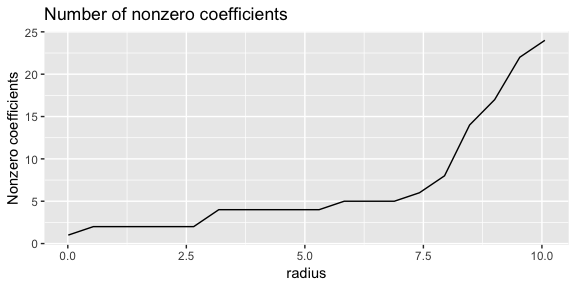Instead using the additional argument type = "path" yields the full coefficient paths for the estimates for all values of the radius. plot(corrected_fit, type = "path")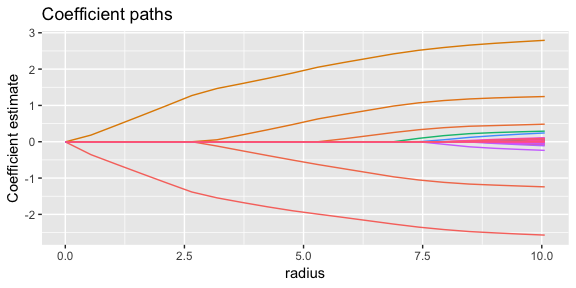## Corrected Lasso for Generalized Linear Models Sorensen, Frigessi, and Thoresen (2015) show how we can also correct for measurement error in the lasso for generalized linear models, using the conditional score method introduced by Stefanski and Carroll (1987), combined with the projected gradient descent algorithm used by Loh and Wainwright (2012) for the corrected lasso for linear models. ### Logistic Regression The corrected lasso for logistic regression is implemented in hdme. To illustrate its use, we start by generating some measurement error data with binomially distributed response. set.seed(323) n <- 100 p <- 50 ll <- create_example_data(n, p, sdU = 0.2, family = "binomial") We get logistic regression by specifying family = "binomial" in the call the fit_corrected_lasso(). corrected_fit <- fit_corrected_lasso(ll$W, ll$y, ll$sigmaUU, family = "binomial")

The plot functions work the same way as for linear regression. By default, the argument type = "nonzero".

plot(corrected_fit)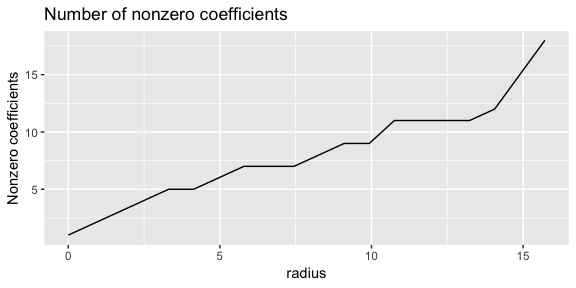Setting type = "path", we get the coefficient values along the regularization parameter $$R$$.

plot(corrected_fit, type = "path")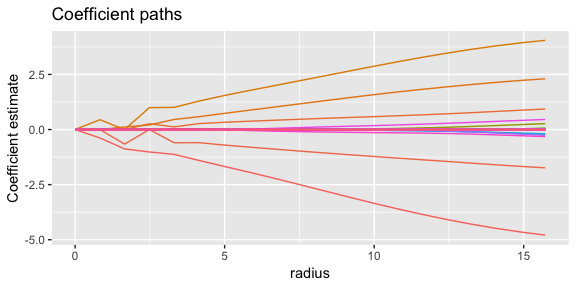### Poison Regression

Poisson regression is also available, by using the argument family = "poisson" to fit_corrected_lasso.

## Model Tuning

The corrected lasso for linear regression has a clearly defined loss function, $L(\beta) = \|y - W\beta\|_{2}^{2} - \beta^{T} \Sigma_{uu} \beta.$ In order to find the optimal value of the regularization parameter $$R$$, we can hence use cross-validation to minimize $$L(\beta)$$. This is implemented in the function cv_corrected_lasso. We illustrate its use below.

For generalized linear models, we are using the conditional score approach, which does not yield a well defined loss function. Hence, cross-validation is not straightforward in these cases.

set.seed(1000)
# Generate example data
ll <- create_example_data(n, p)
# Run lasso with cross-validation
cv_corrected_fit <- cv_corrected_lasso(W = ll$W, y = ll$y, sigmaUU = ll$sigmaUU) The result is a list of class cv_corrected_lasso. class(cv_corrected_fit) #>  "cv_corrected_lasso" The print below shows all the elements of the resulting list. str(cv_corrected_fit) #> List of 5 #>$ cv        :'data.frame':  100 obs. of  5 variables:
#>   ..$radii : num [1:100] 0.0115 0.128 0.2444 0.3609 0.4773 ... #> ..$ mean_loss: num [1:100] 8.74 8.45 8.16 7.88 7.59 ...
#>   ..$sd_loss : num [1:100] 5.54 5.33 5.09 4.86 4.64 ... #> ..$ upper_1se: num [1:100] 10.5 10.13 9.77 9.41 9.06 ...
#>   ..$lower_1se: num [1:100] 6.99 6.76 6.55 6.34 6.13 ... #>$ radius_min: num 8.98
#>  $loss_min : num -3.79 #>$ radius_1se: num 7.11
#>  $loss_1se : num -3.27 #> - attr(*, "class")= chr "cv_corrected_lasso" The element cv contains all the details of the cross-validation runs. • radii contains all the constraint radii $$R$$ that were used. • mean_loss contains the mean of the loss function at the given radius over all folds. • sd_loss gives the standard deviation of the loss at the given radius. • upper_1se and lower_1se contain the upper and lower standard error of mean_loss at the given radius. Next, loss_min gives the minimum of the mean loss, and radius_min is the corresponding radius. loss_1se gives the smallest loss within one standard error of loss_min, and radius_1se is a smallest radius given a loss less than or equal to loss_1se. The result of performing cross-validation can be illustrated using the plot function. It shows the cross-validated loss over the grid of radii. $$R_{min}$$ and $$R_{1se}$$ are shown with labels, and the corresponding loss as horizontal red lines. plot(cv_corrected_fit)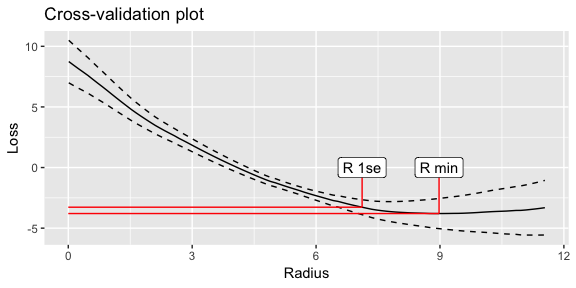Having used cv_corrected_lasso to find the right value of the constraint parameter $$R$$, we can use fit_corrected_lasso on this single value. The snippet below shows how to compute the solution using $$R_{1se}$$. corrected_fit <- fit_corrected_lasso(ll$W, ll$y, ll$sigmaUU, radii = cv_corrected_fit$radius_1se) The final parameter estimates can be found in corrected_fit$betaCorr.

str(corrected_fit)
#> List of 2
#>  $betaCorr: num [1:50, 1] -2.69 -1.13 0 1.2 1.73 ... #>$ radii   : num 7.11
#>  - attr(*, "class")= chr "corrected_lasso"

# Matrix Uncertainty Selector

The Matrix Uncertainty Selector (MUS) was introduced by Rosenbaum and Tsybakov (2010) as a modification of the Dantzig Selector for data with measurement error. The key insight behind the MUS, is that when $$X$$ is measured with error, the true coefficient vector $$\beta$$ may not be part of the feasible set, even when $$\lambda$$ is set to its theorically optimal value. The reason is that $$\lambda$$ is a bound on the residual of the linear model, while in the case of measurement error, a bound on $$\delta$$ the measurement error matrix $$U$$ is also needed. The MUS is defined as the optimization problem $\text{minimize } ~ \|\beta\|_{1}, ~ \text{ subject to } ~ (1/n)\| W^{T} (y - W\beta)\|_{\infty} \leq \lambda + \delta \|\beta\|_{1}.$ Note that the MUS does not require an estimate of the measurement error covariance matrix $$\Sigma_{uu}$$. This might be a practical advantage in some cases, when an estimate of $$\Sigma_{uu}$$ is hard to obtain.

The MUS can be converted to a linear programming problem (Sorensen et al. (2018)). The hdme package contains a function fit_mus for computing the Matrix Uncertainty Selector. It uses Rglpk (Theussl and Hornik (2019)) for solving the underlying linear program. In order to illustrate fit_mus, we generate some example data with measurement error.

set.seed(1)
# Number of samples
n <- 1000
# Number of covariates
p <- 50
# Generate data
ll <- create_example_data(n, p, sdU = 0.2)

We provide the measurement matrix and the response. The solution is computed over a grid of $$\delta$$ values, and with $$\lambda$$ set to the cross-validated optimum for the lasso, as chosen by cv.glmnet in the glmnet package. The returned object is a list with class gmus, which stands for generalized matrix uncertainty selector.

mus_fit <- fit_mus(ll$W, ll$y)
class(mus_fit)
#>  "gmus"
str(mus_fit)
#> List of 6
#>  $intercept : num [1:26] 0.0195 0.0195 0.0195 0.0195 0.0195 ... #>$ beta        : num [1:50, 1:26] -1.89 -0.976 0.438 0.92 1.917 ...
#>  $family : chr "gaussian" #>$ delta       : num [1:26] 0 0.02 0.04 0.06 0.08 0.1 0.12 0.14 0.16 0.18 ...
#>  $lambda : num 0.0257 #>$ num_non_zero: num [1:26] 13 3 8 8 7 3 3 3 2 2 ...
#>  - attr(*, "class")= chr "gmus"

The default plot method shows the number of nonzero coefficients along the grid of $$\delta$$ values chosen by the algorithm.

plot(mus_fit)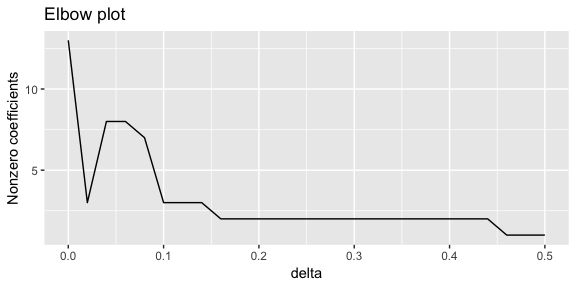According to the “elbow rule” (Rosenbaum and Tsybakov (2010)), a final value of $$\delta$$ can be chosen where the curve starts to level off. This is not always easy in practice, but in the plot above, a value between $$0.05$$ and $$0.1$$ may be reasonable. Choosing $$1.0$$, we can call the algorithm again, but this time setting the argument delta = 0.1.

mus_fit <- fit_mus(ll$W, ll$y, delta = 0.1)

Since only one value of $$\delta$$ was provided, the plot method will now show all the estimated coefficients, rather than the number of nonzero coefficients, as in the previous plot.

plot(mus_fit)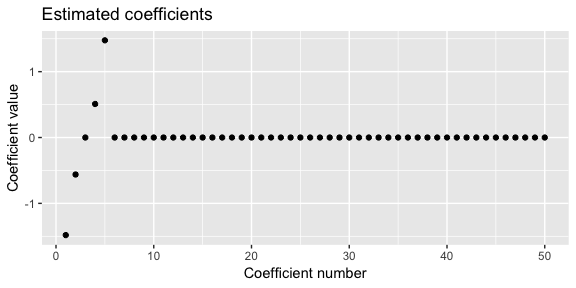## Generalized Matrix Uncertainty Selector

The Generalized Matrix Uncertainty Selector (GMUS) is an extension of the MUS to generalized linear models, introduced by Sorensen et al. (2018). It is defined as the solution to the optimization problem

$\text{minimize } ~ \|\beta\|_{1}, ~ \text{ subject to } ~ (1/n)\|W^{T} (y - \mu(W \beta))\|_{\infty} \leq \lambda + \sum_{r=1}^{R}\delta^{r}(r! \sqrt{n})^{-1} \|\beta\|_{1}^{r} \|\mu^ {(r)}(W\beta)\|_{2},$ where $$\mu(\cdot)$$ is the vector valued mean function of the generalized linear model and $$\mu^{(r)}(\cdot)$$ is its $$r$$th derivative. $$R$$ is a parameter controlling the number of Taylor expansion terms which are included. When $$R\to\infty$$, the true solution is a member of the feasible set given that the bounds $$(1/n)\|W^{T}\epsilon\|_{\infty} \leq \lambda$$ and $$\|U\|_{\infty} \leq \delta$$ hold. In practice, we set $$R=1$$ for computational reasons. When $$R=1$$, the GMUS can be solved using a sequence of linear programming problems on the same form as the MUS, with an iterative reweighing algorithm.

The fit_gmus function in the hdme package implements the GMUS for $$R=1$$, which is defined as $\text{minimize } ~ \|\beta\|_{1}, ~ \text{ subject to } ~ (1/n)\|W^{T} (y - \mu(W \beta))\|_{\infty} \leq \lambda + \delta n^{-(1/2)} \|\beta\|_{1} \|\mu^ {(1)}(W\beta)\|_{2}.$ As the MUS, the GMUS does not require an estimate of $$\Sigma_{uu}$$.

We illustrate fit_gmus using logistic regression. First, we generate some sample data.

set.seed(323)
n <- 100
p <- 50
ll <- create_example_data(n, p, sdU = 0.2, family = "binomial")
gmus_fit <- fit_gmus(ll$W, ll$y, family = "binomial")

The returned object is again a list with class gmus.

class(gmus_fit)
#>  "gmus"
str(gmus_fit)
#> List of 6
#>  $intercept : num [1:26] -0.588 -0.322 -0.257 -0.225 -0.204 ... #>$ beta        : num [1:50, 1:26] -3.15 -1.33 0.91 1.66 2.74 ...
#>  $family : chr "binomial" #>$ delta       : num [1:26] 0 0.02 0.04 0.06 0.08 0.1 0.12 0.14 0.16 0.18 ...
#>  $lambda : num 0.00926 #>$ num_non_zero: num [1:26] 19 13 7 5 11 10 8 6 7 7 ...
#>  - attr(*, "class")= chr "gmus"

The model fitting works the same way as for the MUS. A $$\lambda$$ value is found by running the lasso with the appropriate link function, and taking the value yielding minimum cross-validation loss. A range of $$\delta$$ values are tried. We can call the plot function to study the behavior.

plot(gmus_fit)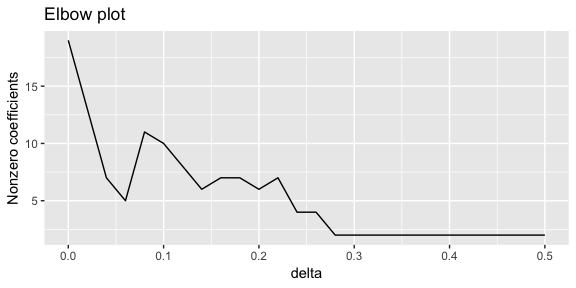Again we see that the number of nonzero coefficients decreases in $$\delta$$. Following the elbow rule, a reasonable choice for $$\delta$$ might here be $$0.1$$. To obtain a final estimate, we might therefore run fit_gmus again with this value.

gmus_fit <- fit_gmus(ll$W, ll$y, delta = 0.1, family = "binomial")

The plot will now show the estimated coefficients.

plot(gmus_fit)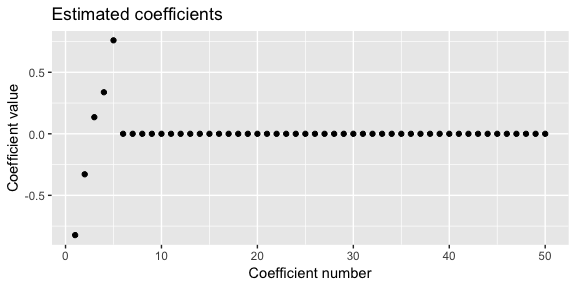### Poisson Regression

The Generalized Matrix Uncertainty Selector is also implemented for Poisson regression. Use the argument family = "poisson" to fit_gmus.

# Generalized Matrix Uncertainty Lasso

A “lasso equivalent” of the Generalized Matrix Uncertainty Selector can also be defined (Rosenbaum and Tsybakov (2010), Sorensen et al. (2018)). We refer to Sorensen et al. (2018) for the details of this algorithm.

This GMU Lasso is implemented in hdme, and can be called with the function fit_gmu_lasso. The snippet below shows its use. The underlying coordinate descent algorithm is implemented in C++ using the RcppArmadillo package (Eddelbuettel and Sanderson (2014)). Both logistic and Poisson regression are supported, with arguments family = "binomial" and family = "poisson", respectively.

set.seed(323)
n <- 100
p <- 50
ll <- create_example_data(n, p, sdU = 0.2, family = "binomial")
gmu_lasso_fit <- fit_gmu_lasso(ll$W, ll$y, family = "binomial")

The returned object is a list with class gmu_lasso.

class(gmu_lasso_fit)
#>  "gmu_lasso"
str(gmu_lasso_fit)
#> List of 6
#>  $intercept : num [1:5] -0.591 -0.283 -0.224 -0.195 -0.177 #>$ beta        : num [1:50, 1:5] -3.325 -1.382 0.897 1.769 2.799 ...
#>  $family : chr "binomial" #>$ delta       : num [1:5] 0 0.05 0.1 0.15 0.2
#>  $lambda : num 0.00926 #>$ num_non_zero: num [1:5] 13 8 5 4 4
#>  - attr(*, "class")= chr "gmu_lasso"

The plotting function gives an elbow plot, which can be used to select the regularization parameter delta.

plot(gmu_lasso_fit)